### Areas of Parallelograms and Triangles - Test Papers

CBSE Test Paper 01

CH-9 Areas of Parallelograms & Triangles

1. The area of the parallelogram ABCD in the figure is :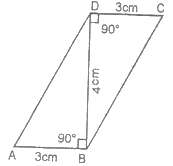1. $12\phantom{\rule{thinmathspace}{0ex}}c{m}^{2}$

2. $10\phantom{\rule{thickmathspace}{0ex}}c{m}^{2}$

3. $9\phantom{\rule{thickmathspace}{0ex}}c{m}^{2}$

4. $15\phantom{\rule{thickmathspace}{0ex}}c{m}^{2}$

2. Find the area of parallelogram in the adjoining figure.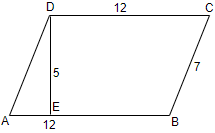1. 60 square feet

2. 48 square feet

3. 1759 feet

4. 84 square feet

3. PQRS is a parallelogram. If X and Y are mid-points of PQ and SR and diagonal SQ is joined, then $ar\left(\parallel \phantom{\rule{thickmathspace}{0ex}}XQRY\right):ar\left(\mathrm{△}QSR\right)$ is equal to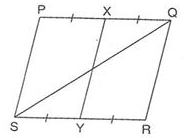1. it is 2 : 1.

2. it is 1 : 2.

3. it is 1 : 1.

4. it is 1 : 4.

4. Points A, B, C, and D are collinear. AB = BC = CD. $XY\parallel AD.$ If P and M lie on XY and $ar\left(\mathrm{△}MCD\right)=7\phantom{\rule{thickmathspace}{0ex}}c{m}^{2},$ then $ar\phantom{\rule{thickmathspace}{0ex}}\left(\mathrm{△}APB\right)\phantom{\rule{thickmathspace}{0ex}}$and $\phantom{\rule{thickmathspace}{0ex}}ar\phantom{\rule{thickmathspace}{0ex}}\left(\mathrm{△}APD\right)$ respectively are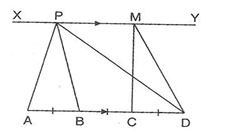1. $7\phantom{\rule{thickmathspace}{0ex}}c{m}^{2},\phantom{\rule{thickmathspace}{0ex}}21\phantom{\rule{thickmathspace}{0ex}}c{m}^{2}.\phantom{\rule{thickmathspace}{0ex}}$

2. $7\phantom{\rule{thickmathspace}{0ex}}c{m}^{2},14\phantom{\rule{thickmathspace}{0ex}}c{m}^{2}.\phantom{\rule{thickmathspace}{0ex}}$

3. $\phantom{\rule{thickmathspace}{0ex}}14\phantom{\rule{thickmathspace}{0ex}}c{m}^{2},14\phantom{\rule{thinmathspace}{0ex}}c{m}^{2}.$

4. $14\phantom{\rule{thickmathspace}{0ex}}c{m}^{2},\phantom{\rule{thinmathspace}{0ex}}21\phantom{\rule{thickmathspace}{0ex}}c{m}^{2}.$

5. If the sum of the parallel sides of a trapezium is 7 cm and distance between them is 4 cm, then area of the trapezium is :

1. $7\phantom{\rule{thinmathspace}{0ex}}c{m}^{2}$

2. $21\phantom{\rule{thickmathspace}{0ex}}c{m}^{2}$

3. $14\phantom{\rule{thickmathspace}{0ex}}c{m}^{2}$

4. $28\phantom{\rule{thickmathspace}{0ex}}c{m}^{2}$

6. Fill in the blanks:

The area of a rhombus is equal to ________ of the product of its two diagonals.

7. Fill in the blanks:

A median of a triangle divides it into ________ triangles of equal areas.

8. In a given figure, it is given that AD || BC. Prove that ar($\mathrm{△}$CGD) = ar($\mathrm{△}$ABG).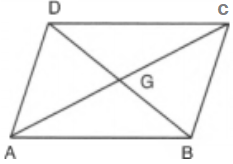9. Is the given figure lie on the same base and between a same parallels. In such a case, write the common base and the two parallels :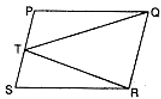10. In Fig., X and Y are the mid-points of AC and AB respectively, QP || BC and CYQ and BXP are straight lines. Prove that ar ($\mathrm{△}$ABP) = ar ($\mathrm{△}$ACQ).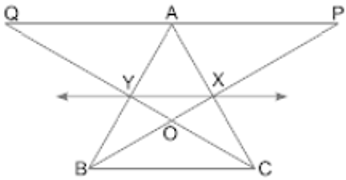11. ABCD is a parallelogram. P is any point on CD. If area($\mathrm{△}$DPA) = 15 cm2 and area ($\mathrm{△}$APC) = 20 cm2, find the area($\mathrm{△}$APB).

12. In figure, AP || BQ || CR. Prove that ar(AQC) = ar(PBR).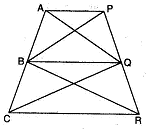13. D and E are mid-points of BC and AD respectively. If area of $\mathrm{△}$ABC = 10 cm2, find area of $\mathrm{△}$EBD.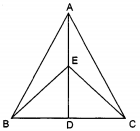14. In the given Fig., X and y are points on the side LN of the triangle LMN such that LX = XY = YN. Through X, a line is drawn parallel to LM to meet MN at Z. Prove that ar ($\mathrm{△}$LZY) = ar (MZYX).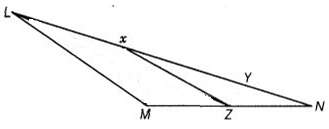15. If AD is a median of a triangle ABC, then prove that triangles ADB and ADC are equal in area. If G is the mid-point of median AD, prove that ar($\mathrm{△}$BGC) = 2ar($\mathrm{△}$AGC).

CBSE Test Paper 01
CH-9 Areas of Parallelograms & Triangles

Solution

1. (a) $12\phantom{\rule{thinmathspace}{0ex}}c{m}^{2}$
Explanation:
Area of parallelogram = Base $×$ Height
= AB $×$ BD
= 3 $×$ 4
= 12 cm2
2. (a) 60 square feet
Explanation:
In the figure, Base AB = 12 feet and Correspongin height DE = 5 feet
Then
Area of parallelogram ABCD = Base $×$ Corresponding height
= AB $×$ DE
= 12 $×$ 5
= 60 square feet
3. (c) it is 1 : 1.
Explanation:
Since parallelogram PQRS and triangle AQS are on the same base QS and between the same parallels, then
$\mathrm{a}\mathrm{r}\left(\mathrm{△}\mathrm{P}\mathrm{Q}\mathrm{S}\right)=\frac{1}{2}×\mathrm{a}\mathrm{r}\left(\parallel gm\mathrm{P}\mathrm{Q}\mathrm{R}\mathrm{S}\right)$              .................(i)
Also, if X and Y are mid-points of PQ and SR and diagonal SQ is joined, then
$\mathrm{a}\mathrm{r}\mathrm{e}\mathrm{a}\left(\parallel gm\mathrm{X}\mathrm{Q}\mathrm{R}\mathrm{Y}\right)=\frac{1}{2}×\mathrm{a}\mathrm{r}\mathrm{e}\mathrm{a}\left(\parallel gm\mathrm{P}\mathrm{Q}\mathrm{R}\mathrm{S}\right)$          ....................(ii)
From eq.(i) and (ii), we get
$\mathrm{a}\mathrm{r}\mathrm{e}\mathrm{a}\left(\mathrm{△}\mathrm{P}\mathrm{Q}\mathrm{S}\right)=\mathrm{a}\mathrm{r}\mathrm{e}\mathrm{a}\left(\parallel gm\mathrm{X}\mathrm{Q}\mathrm{R}\mathrm{Y}\right)$
$⇒$ $\mathrm{a}\mathrm{r}\mathrm{e}\mathrm{a}\left(\parallel gm\mathrm{X}\mathrm{Q}\mathrm{R}\mathrm{Y}\right):\mathrm{a}\mathrm{r}\mathrm{e}\mathrm{a}\left(\mathrm{△}\mathrm{P}\mathrm{Q}\mathrm{S}\right)=1:1$
4. (a) $7\phantom{\rule{thickmathspace}{0ex}}c{m}^{2},\phantom{\rule{thickmathspace}{0ex}}21\phantom{\rule{thickmathspace}{0ex}}c{m}^{2}.\phantom{\rule{thickmathspace}{0ex}}$

Explanation:

Since triangles MCD and PAB are on the same base and between the same parallels, then
$\mathrm{a}\mathrm{r}\mathrm{e}\mathrm{a}\left(\mathrm{△}\mathrm{M}\mathrm{C}\mathrm{D}\right)=\mathrm{a}\mathrm{r}\mathrm{e}\mathrm{a}\left(\mathrm{△}\mathrm{P}\mathrm{A}\mathrm{B}\right)=7$ sq. cm
Now, since AB = BC = CD, then
AB = $\frac{1}{3}$AD and BC = $\frac{2}{3}$AD
Then, $\mathrm{a}\mathrm{r}\mathrm{e}\mathrm{a}\left(\mathrm{△}\mathrm{A}\mathrm{P}\mathrm{B}\right)=\frac{1}{3}×\mathrm{a}\mathrm{r}\mathrm{e}\mathrm{a}\left(\mathrm{△}\mathrm{A}\mathrm{P}\mathrm{D}\right)$
$⇒$ $\frac{1}{3}×\mathrm{a}\mathrm{r}\mathrm{e}\mathrm{a}\left(\mathrm{△}\mathrm{A}\mathrm{P}\mathrm{D}\right)=7$ sq. cm
$⇒$  $\mathrm{a}\mathrm{r}\mathrm{e}\mathrm{a}\left(\mathrm{△}\mathrm{A}\mathrm{P}\mathrm{D}\right)=21$ sq. cm

5. (c) $14\phantom{\rule{thickmathspace}{0ex}}c{m}^{2}$

Explanation:

Given: Sum of the parallel sides of a trapezium is 7 cm and distance between them is 4 cm.

Area of the trapezium = $\frac{1}{2}$(Sum of parallel sides) x Height

$\frac{1}{2}$x 7 x 4

= 14 cm2

6. $\frac{1}{2}$

7. two

8. Since $\mathrm{△}$ADC and $\mathrm{△}$ADB are on the same base AD and between the same parallels AD and BC.
ar($\mathrm{△}$ADC) = ar($\mathrm{△}$ADB)
$⇒$ ar($\mathrm{△}$ADC) - ar($\mathrm{△}$ADG) = ar($\mathrm{△}$ADB) - ar($\mathrm{△}$ADG)
$⇒$ ar($\mathrm{△}$CGD) = ar($\mathrm{△}$ABG)

9. $△$TRQ and parallelogram SRQP lie on the same base RQ and between the same parallels RQ and SP.

10. From the given figure it is clear that X and Y are the mid-points of AC and AB respectively.
Therefore,  XY $\parallel$ BC
Since $\mathrm{△}$BYC and $\mathrm{△}$BXC are on the same base BC and between the same parallels XY and BC, we can write
ar ($\mathrm{△}$BYC) = ar ($\mathrm{△}$BXC)
$⇒$ar ($\mathrm{△}$BYC) - ar ($\mathrm{△}$BOC) = ar ($\mathrm{△}$BXC) - ar ($\mathrm{△}$BOC)
$⇒$ ar ($\mathrm{△}$BOY) = ar ($\mathrm{△}$COX)
$⇒$ar ($\mathrm{△}$BOY) + ar ($\mathrm{△}$XOY) = ar ($\mathrm{△}$COX) + ar ($\mathrm{△}$XOY)
$⇒$ar ($\mathrm{△}$BXY) = ar ($\mathrm{△}$CXY) .......................................(i)
Since quadrilaterals XYAP and XYQA are on the same base XY and between the same parallels XY and PQ, we have
ar(XYAP) = ar (XYQA) .........................................(ii)
On adding (i) and (ii), we obtain
ar ($\mathrm{△}$BXY) + ar (XYAP) = ar ($\mathrm{△}$CXY) + ar (XYQA)
$⇒$ar ($\mathrm{△}$ABP) = ar ($\mathrm{△}$ ACQ)

Hence proved.

11.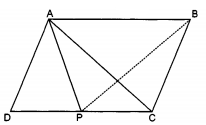From the given figure it is clear that area ($\mathrm{△}$ADC) = area ($\mathrm{△}$ADP)+ area($\mathrm{△}$APC)  = 15 + 20 = 35 cm2
Now in parallelogram opposite sides are equal i.e., CD = AB,

Also AB $\parallel$ CD.
Now, we know that triangles having same base and lie with the same parallels have the same area.

$\therefore$  area of $\mathrm{△}$ABP = area of $\mathrm{△}$ADC =  35 cm2

12. Given : AP || BQ || CR
To Prove : ar($\mathrm{△}$AQC) = ar($\mathrm{△}$PBR)
Proof : ar($\mathrm{△}$BAQ) = ar($\mathrm{△}$BPQ) . . . .[$\mathrm{△}$s on the base BQ and between the same parallels BQ and AP] . . . (1)
ar($\mathrm{△}$BCQ) = ar($\mathrm{△}$BQR) . . . .[$\mathrm{△}$s on the base BQ and between the same parallels BQ and CR] . . . (2)
ar($\mathrm{△}$BAQ) + ar($\mathrm{△}$BCQ) = ar($\mathrm{△}$BPQ) + ar($\mathrm{△}$BQR) . . . [Adding corresponding sides of (1) and (2)]
$⇒$ ar($\mathrm{△}$AQC) = ar($\mathrm{△}$PBR)

13. It is given that D is the midpoint of BC, therefore we can say that AD is the median.Now, Area of $\mathrm{△}$ABD = $\frac{1}{2}$ $×$ area of $\mathrm{△}$ABC   ............[Median divides a triangle into two triangles of the equal area]
Thus, Area of $\mathrm{△}$ABD = $\frac{1}{2}×10$ cm2 = 5 cm2
Again, BE is the median of $\mathrm{△}$ABD.
$\therefore$ Area of $\mathrm{△}$EBD = $\frac{1}{2}$ $×$ area of $\mathrm{△}$ABD
= $\frac{1}{2}×5$ = 2.5 cm2

14. From the given figure,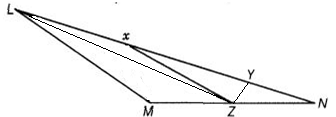it is clear that $\mathrm{△}$LXZ and $\mathrm{△}$MXZ  (adding x and M) lie on the same base XZ and between the same parallels XZ and LM.
$\therefore$ ar($\mathrm{△}$LXZ) = ar($\mathrm{△}$MXZ)
Adding ar($\mathrm{△}$XYZ) on both sides, we obtain
ar($\mathrm{△}$LXZ) + ar($\mathrm{△}$XYZ) = ar($\mathrm{△}$MXZ) + ar($\mathrm{△}$XYZ)
$⇒$ar ($\mathrm{△}$LYZ) = ar(MZYX)
Hence proved

15.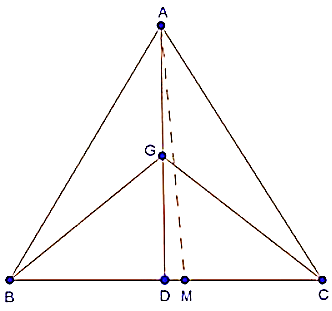Draw AM $\perp$ BC.
Since, AD is the median of $\mathrm{△}$ABC,
$\therefore$ BD = DC
$⇒$ BD $×$ AM = DC $×$ AM
$⇒$ $\frac{1}{2}$(BD $×$ AM) = $\frac{1}{2}$(DC $×$ AM)
$⇒$ ar($\mathrm{△}$ABD) = ar($\mathrm{△}$ACD) .....(i)
In $\mathrm{△}$BGC, GD is the median,
$\therefore$ ar($\mathrm{△}$BGD) = ar($\mathrm{△}$CGD) ....(ii)
In $\mathrm{△}$ACD, CG is a median,
$\therefore$ ar($\mathrm{△}$AGC) = ar($\mathrm{△}$CGD) .....(iii)
From (ii) and (iii), we have,
ar($\mathrm{△}$BGD) = ar($\mathrm{△}$AGC)
But, ar($\mathrm{△}$BGC) = 2ar($\mathrm{△}$BGD)
$\therefore$ ar($\mathrm{△}$BGC) = 2 ar($\mathrm{△}$AGC)